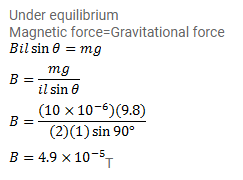# A straight, horizontal wire of mass 10mg and length 1.0m

Question:

A straight, horizontal wire of mass $10 \mathrm{mg}$ and length $1.0 \mathrm{~m}$ carries a current of $2.0 \mathrm{~A}$. What minimum magnetic field B should be applied in the region so that the magnetic force on the wire may balance its weight?

Solution: